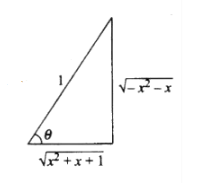# Find the real solution of the equation

Question:

Find the real solution of the equation

$\tan ^{-1} \sqrt{x(x+1)}+\sin ^{-1} \sqrt{x^{2}+x+1}=\frac{\pi}{2}$

Solution:

Given equation,

$\tan ^{-1} \sqrt{x(x+1)}+\sin ^{-1} \sqrt{x^{2}+x+1}=\frac{\pi}{2}$

$\tan ^{-1} \sqrt{x(x+1)}=\frac{\pi}{2}-\sin ^{-1} \sqrt{x^{2}+x+1}$

$=\cos ^{-1} \sqrt{x^{2}+x+1}$$=\tan ^{-1} \frac{\sqrt{-x^{2}-x}}{\sqrt{x^{2}+x+1}}$ (From figure)

$\sqrt{x(x+1)}=\frac{\sqrt{-x^{2}-x}}{\sqrt{x^{2}+x+1}}$

$x^{2}+x=0$

$x=0,-1$

Hence, the real solutions of the given trigonometric equation are 0 and -1.